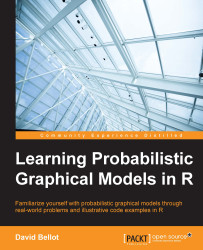•#### Learning Probabilistic Graphical Models in R#### Overview of this book

Probabilistic graphical models (PGM, also known as graphical models) are a marriage between probability theory and graph theory. Generally, PGMs use a graph-based representation. Two branches of graphical representations of distributions are commonly used, namely Bayesian networks and Markov networks. R has many packages to implement graphical models. We’ll start by showing you how to transform a classical statistical model into a modern PGM and then look at how to do exact inference in graphical models. Proceeding, we’ll introduce you to many modern R packages that will help you to perform inference on the models. We will then run a Bayesian linear regression and you’ll see the advantage of going probabilistic when you want to do prediction. Next, you’ll master using R packages and implementing its techniques. Finally, you’ll be presented with machine learning applications that have a direct impact in many fields. Here, we’ll cover clustering and the discovery of hidden information in big data, as well as two important methods, PCA and ICA, to reduce the size of big problems.
Learning Probabilistic Graphical Models in RCreditswww.PacktPub.comPrefaceFree Chapter
Probabilistic ReasoningExact InferenceLearning ParametersBayesian Modeling – Basic ModelsApproximate InferenceBayesian Modeling – Linear ModelsProbabilistic Mixture ModelsAppendixIndex## Summary

In this chapter we saw how to compute the parameters of a graphical model by using the maximum likelihood estimation.

The reader should note however that this approach is not Bayesian and could be improved by setting prior distributions over the parameters of the graphical models. This could be used to include more domain knowledge and help in obtaining better estimations.

When the data is not fully observed and variables are hidden, we learned how to use the very powerful EM algorithm. We also saw a full implementation of a learning algorithm in R for a fully observed graph.

We would like, at this point, to encourage the reader to use the ideas presented in this chapter to extend and improve his or her own learning algorithms. The most important requirement when doing machine learning is to focus on what is not working. From a dataset, any algorithm will, at some point, extract some information. However, when one focuses on the errors in an algorithm and where it does not work, one...